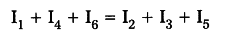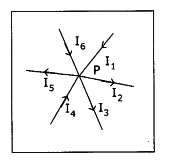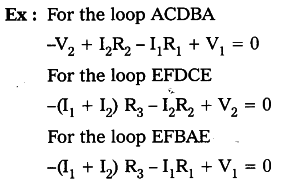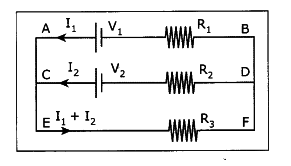# Explain Kirchhoffs laws with examples

Kirchhoffs laws :

1. The junction law : At any junction point in a circuit where the current can divide, the sum of the currents in the junction must equal to the sum of the currents leaving the junction.
This means that there is no accumulation of electric charges at any junction in a circuit.
Ex : ‘P’ is the junction
I _{1} I _{4} and I _{6} are the currents into the junction.
I _{2},I _{3} and I _{5} are the currents leaving the junction.
According to Kirchhoffs junction law1. The loop law : The algebraic sum of the increases and decreases in potential difference across various components of a closed circuit loop must be zero.